Olympiad Test Level 2: Number’s Magic- 1

# Olympiad Test Level 2: Number’s Magic- 1

Test Description

## 10 Questions MCQ Test GK Olympiad for Class 4 | Olympiad Test Level 2: Number’s Magic- 1

Olympiad Test Level 2: Number’s Magic- 1 for Class 4 2023 is part of GK Olympiad for Class 4 preparation. The Olympiad Test Level 2: Number’s Magic- 1 questions and answers have been prepared according to the Class 4 exam syllabus.The Olympiad Test Level 2: Number’s Magic- 1 MCQs are made for Class 4 2023 Exam. Find important definitions, questions, notes, meanings, examples, exercises, MCQs and online tests for Olympiad Test Level 2: Number’s Magic- 1 below.
 1 Crore+ students have signed up on EduRev. Have you?
Olympiad Test Level 2: Number’s Magic- 1 - Question 1

### Find the missing number, if same rule is followed in all the three figures.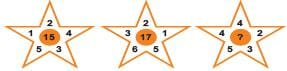Detailed Solution for Olympiad Test Level 2: Number’s Magic- 1 - Question 1

The rule followed here is sum of all the numbers given on the sides is equal to the number given in the centre.
In figure 1, sum of numbers = 1+2+4+3+5 = 15
In figure 2, sum of numbers = 3+2 +1+5+6 = 17
Therefore, the centre number is equal to the sum of numbers = 4+4 +2+3+5 = 18

Olympiad Test Level 2: Number’s Magic- 1 - Question 2

### The given figure is made up of identical squares. Find the perimeter of the given figure.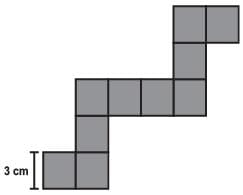Detailed Solution for Olympiad Test Level 2: Number’s Magic- 1 - Question 2

Perimeter is calculated by adding the length of the sides surrounding a shape.
Here, we have 22 sides, if we add all the sides of identical sides.
Each side length = 3 cm
Therefore, Perimeter = 22*3 = 66 cm

Olympiad Test Level 2: Number’s Magic- 1 - Question 3

### On the basis of the figure, which of the following statements are true?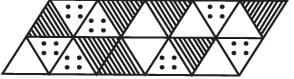Detailed Solution for Olympiad Test Level 2: Number’s Magic- 1 - Question 3

In the given figure, total number of triangles = 20
Number of triangles with lines = 7
Number of triangles with dots = 7
Number of blank triangles = 6
Therefore, option B is correct, as fraction of triangles with dots = 7/20.

Olympiad Test Level 2: Number’s Magic- 1 - Question 4

How many lines of symmetry does a square have?

Detailed Solution for Olympiad Test Level 2: Number’s Magic- 1 - Question 4

A square has 4 lines of symmetry.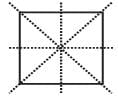Olympiad Test Level 2: Number’s Magic- 1 - Question 5

If there are 4 goats and 3 cows, then how many animal legs are there?

Detailed Solution for Olympiad Test Level 2: Number’s Magic- 1 - Question 5

Goats and cows have 4 legs.
Total legs= (4+3)*4= 28 legs

Olympiad Test Level 2: Number’s Magic- 1 - Question 6

Which diagram correctly represents relationship among: Apples, Oranges, Fruits?

Detailed Solution for Olympiad Test Level 2: Number’s Magic- 1 - Question 6

As apples and oranges come under fruits, option A correctly depicts their relationship.

Olympiad Test Level 2: Number’s Magic- 1 - Question 7

Which expression is odd one in the given options?

Detailed Solution for Olympiad Test Level 2: Number’s Magic- 1 - Question 7

In all the options, after solving we get 23, except for B where answer is 0.

Olympiad Test Level 2: Number’s Magic- 1 - Question 8

Disha is facing towards north. She makes 3/4 turn to her right and then 1/2 turn in clockwise direction. Now, if she wants to face towards the temple, then what turn will she make from the given options?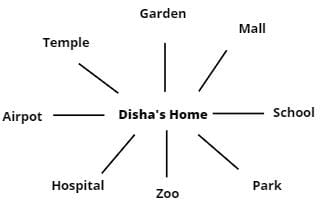Detailed Solution for Olympiad Test Level 2: Number’s Magic- 1 - Question 8

Disha is facing towards north i.e. she is facing towards garden. After making 3/4 turn to her right and 1/2 turn in clockwise direction, she will face towards school. Now, if she wants to face towards temple then she will have to make 5/8 turn clockwise.

Olympiad Test Level 2: Number’s Magic- 1 - Question 9

What will be the final answer if we mix 72l 95 ml and 12 l 936 ml?

Detailed Solution for Olympiad Test Level 2: Number’s Magic- 1 - Question 9

We should add quantities with the same unit of measurement.
Here adding 936 ml + 95 ml = 1031 ml = 1l 31 ml
Now adding litre quantities = 72+12+1 = 85l
Therefore, final answer is 85l 31 ml.

Olympiad Test Level 2: Number’s Magic- 1 - Question 10

The below pictograph shows how many bananas were sold in 3 weeks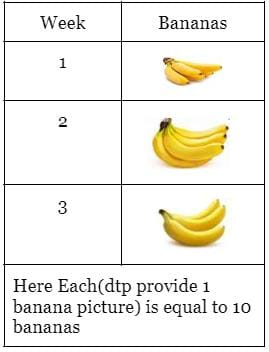How many bananas were sold in week 2?

Detailed Solution for Olympiad Test Level 2: Number’s Magic- 1 - Question 10

Here, Bananas sold in week  = 6*10 = 60 (as each banana is equal to 10 bananas)

## GK Olympiad for Class 4

48 tests
 Use Code STAYHOME200 and get INR 200 additional OFF Use Coupon Code